﻿ Bell CHSH inequality violation in polarized photons and quantum entanglement are useless, illusion

# Quantum faster-than-light entanglement by Bell inequality violation is useless and illusion.

## Photon is a particle ?

Bell inequality violation can be explained by classical electromagnetic waves!.

The photon particle is said to exist. But the interference of a single photon with itself can be explained only by the wave nature of the electromagnetic (E-M) waves, NOT by the photon particle.

(Fig.1) Photon is particle or wave ?According to QED, a single photon must be a point particle.
But in various real experiments, they often use the classical electromagnetic waves such as left-, right-circularly, vertically, and horizontally polartized lights.

Though they clearly use the property specific to the classical lights, they always try to express them as a photon particle in some papers or something.

As shown in Fig.1, if the light is a point particle, how can it be polarized or interact with other particles ?
So the concept of photon "point" particle is impossible.

## "Entangled" photon pair is just classical "polarized" lights.

(Fig.2) Entangled photon pair ?Entangled photon pair is often created by "parametric down-conversion".
In this process, a photon of wavelength λ enters some crystal, and is split into a pair of photons of wavelength 2λ.
So the total energy is conserved. (= their frequency becomes half ).
( If photon is a particle, it can be split so easily ?? )

One of the produced photon pair is horizontally polarized, and another is vertically polarized.
Though this photon pair is clearly classical electromagnetic waves, they always use the word of a "photon".

## If photon is a particle, "interference" is impossible.

(Fig.3) Photon "point" particle can interfere with each other ?Suprisingly, photon "point" particle can interfere with each other, according to their experiments.
In Fig.3 upper, due to the destructive interference between photons, they vanish !.
Conversely, in Fig.3 lower, due to the constructive interference, the number of photons (= amplitude ) has increased, they insist.
But as shown in Fig.3, these interferences prove that what they call "photon" is clearly classical waves.

## Single photon detector just sees photoelectric effect ( NOT a photon ).

(Fig.4) Single photon detector shows photon is particle ?First, we cannot generate single photon directly.
By weakening coherent laser and seeing their electric current signal at photon detector, we only estimate their existence.
This detector cannot always detect photon ( < 60% ), when it enters, and due to dead time, cannot discriminate between a single and two photons.

## Detection "threshold" at detector causes a illusory "photon".

Of course, as we cannot see directly a single photon, this photon's concept is only speculation.
Repeating photoelectric effect, photon detector increases electric current signals from a single photon.
Considering light loss in these processes, it is natural that we think photon detector can show current signal, when the light intensity is above some threshold.

## A single photon can be in the "superposition" in two different paths !?

(Fig.5) Mach-Zehnder interferometer.In the Mach-Zehnder interferometer, when one photon is split into the two different paths at the beam splitter (= BS1 ), we can detect one photon only in one of the two paths at the same time (= Fig.5 ).
( This means that this single photon particle is NOT split into two, according to the quantum mechanical interpretation. )

(Fig.6) Interference of a single photon.But the interference of electromagnetic waves from these two different paths is actually observed, though a single photon exists only in one of the two paths. It is amazing!
It is said that this strange phenomenon is caused by the superposition of one photon in the two different paths.
But is it really so?
This "superposition" has NO reality, because it is equal to "many fantasy worlds". (= world A and world B in this case. )

## Photon = classical lights, which is split at splitter.

(Fig.7) Photon = classical electromagnetic waves !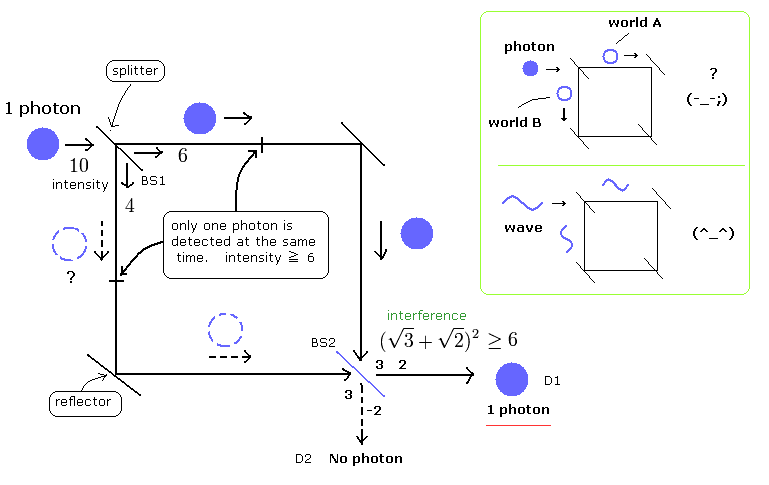Suppose the photon is electromagnetic wave (E-M wave), which is detected as one photon only when the intensity of the E-M wave is above some threshold.
For example, the first light intensity is 10, then it is split by the beam splitter ( BS1 ) into 6 + 4 waves.

We suppose the photon detector can detect it when the light intensity is 6 as a single photon.
So 10 is detected as one photon, not 2 photons (=12).
But the split photon (waves, 6 & 4) can interfere with each other.

And the 4 side is not detected, only 6 side is detected as a photon.
So we can explain the "strange" single photon interference by the combination of the electromagnetic wave and detection threashold !

## Detection threshold + classical light = superposition of a photon.

For example, in case of the 50/50 beamsplitter ( BS1 ), if the E-M waves is split into 5 + 5, no photons can be detected. (This case is not recognized at all, so it can be ignored. )
We can recognize the photon existence only when we detect it as a photon.
50/50 beamsplitter divides unpolarized electromagnetic waves into about half on average.
Depending on the polarization and frequency of the light at the beamsplitter, this probability changes.

(Fig.8) Beam splitter 2 ( BS2 ).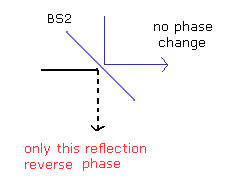After splitting at the beam splitter 1 (BS1), both the electromagnetic waves are split into almost half also by the beam splitter 2 ( BS2 ).
( For example, 6 → 3 + 3, 4 → 2 + 2 )

In this experiment, only one side reflection is supposed to reverse light phase at the beam splitter 2 (BS2), as shown above.
( The transmitted lights are not changed. )

So at the photon detector 1 (D1), the light amplitude is increased by constructive inteference, and can be recognized as a photon.
Because the light intensity at D1 always reaches "6" by the inteference.
(The light amplitude is a square root of the light intensity.).

## Interference + detection threshold causes an illusory single photon.

[ One photon "always" detected at D1 by the interference. ]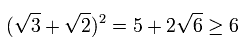On the other hand, at the D2 detector, the light amplitudes from the two paths cancel each other, so it can not be detected as a photon.
( The light intensity at D2 side does not reach "6". )

[ At D2, no photon is detected by the interference. ]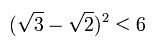Of course, also when the first light "10" is split into 5 + 5 at the beam splitter 1, this result is the same as the above.
In this case the light intensity "5" is split into 2.5 + 2.5, so at D1 side,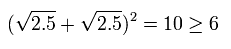And at D2 side,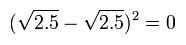In these cases, the total energies are conserved. ( 10 → 10 ).
As a result, we can explain all these phenomena by classical electromagnetic wave model.
So they don't need the concept like "photon particles" at all.

## Delayed choice experiment can be explained by classical lights.

(Fig.9) Delayed choice experiment.In the delayed choice experiment, after a single photon has passed through the beam splitter 1 (= BS1 ), that single photon has entered into one of two paths.
Actually, by the photon detector, only one photon is detected ( for example, at D2 in the upper figure ).

What will happen, when we insert the beam splitter 2 (= BS2 ) after the photon has passed BS1 ?
Surprisingly, in this case, the electromagnetic waves suddenly appear in the two different paths, and interfere with themselves ! (= right panel of the upper figure. )

So we can choose the past event (= particle or wave ) from the future (= delayed choice ) !
Of course, in this real world, it is impossible.
So as I said above, the concept of a single photon itself is wrong.
( Unfortunately, ordinary people do not know about these strange things related to "photon" well . )

## Photon spin "1" is artificial definition, NO reality.

(Fig.10) Photon spin is really " 1 " ?One photon is said to have "spin" ±1. What in the world is the "photon spin 1"?
The ordinary textbooks say that the photon spin ±1 correspond to the electromagnetic (E-M) waves with left and right circular polarization.
And the "linear" polarization corresponds to the superposition of these two states.

But this superposition state is very strange and difficult to imagine.
The quantum mechanics often uses a mathematical trick like this.
Then we can take this convenient word "superposition" in every situation arround us!
(e.g. when some things in different states are only mixed, when one thing is "vibrating" very fast, when one thing only keeps still....).
This means that the superposition itself is meaningless.

(Fig.11) Fine-structure = "photon" spin 1 ??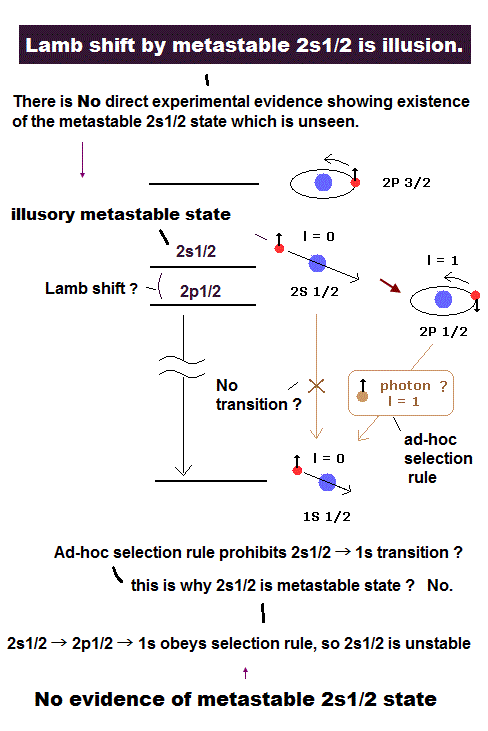To begin with, how this photon spin 1 was discovered ?
In 1910's, the fine structure of the hydrogen atom meant the relativistic energy difference between the 2S and 2P states of Bohr-Sommerfeld model.
But after the electron spin appeared, the interpretation of this fine structure was changed to the spin-orbital interaction ( = the energy difference between 2P1/2 and 2P3/2 of the hydrogen atom. See this page. )

"Accidentally" this value coincided with that of the Bohr-Sommerfeld model !
So the "unnecessary" transition ( = 2S ---> 1S) needs to be forbidden to explain the spectrum results of the hydrogen with electron spin.
Then the selection rule (2S --x--> 1S) was introduced, and they made the photon spin 1 which probably causes this selection rule.
This means that the photon spin 1 is only an artificial thing.

## Photon = point particle ? → Photoelectric effect is impossible.

(Fig.12) Point particle = photoelectric effect ??It is said that the photoelectric effect needs the concept like "photon particle". But is it really so?
The electrons are always accompanied by the de Broglie's waves around them. And it synchronizes with the electron's momentum. So we must consider the de Broglie's waves rather than the very small point-like electron in case of the photoelectric effect or the Compton effect.
Actually, circularly or linearly polarized electromagnetic waves are emitted from exicited atoms in the experiments.
And Willis Lamb himself, who was great experimentalist dealing with the light, did NOT believe photon particle.

Recent interesting studies indicate that a single photon consists of electromagnetic waves ( in both two slits ) in the weak measurements.
(Science 2011, 332, 1170, S.Kocsis et al., Nature 2011, 474, 188, J.S.Lundeen et al.)

## Light frequency = c / λ = wave ( NOT particle ) nature.

(Fig.13) Frequency = photon particle is oscillating ?The ordinary textbooks often say that the relation between the photoelectric effect and the wave's frequency shows the particle's nature of the photon.
First, the frequency itself is based on the electromagnetic wave nature !
Second, when we heat the air or the water which give rise to "sound wave" or "wave", the frequency of each particle in those waves is increased.
So it is quite natural that the energy is related to the frequency.
( Frequency, amplitude = wave nature ! )

In the Compton effects, if the light is a particle, its velocity would change instead of its frequency, when its momentum changes.
As I said above, the frequency itself is based on wave nature.

## Photon "particle" causes "illusory" expanding universe.

(Fig.14) "Convenient" photon can pass through dark energy, matter, Higgs "uninjured"?As shown on this page, photon particle needs to have strange ability to go through very crowded space "uninjured" for very long time.
Redshift by expanding universe is thought to be caused by the tired light, in which the light loses energy while it is going through the space.

The shining duration from supernova is more elongated from farther stars.
You can imagine this reason from the difference between "marathon" and "100-meter race".
And the concept of photon particle is the origin of many-world interpretation and virtual photon, which violates relativity. ( See this page. )

## Faster-than-light quantum entanglement or spooky action expressed by Bell inequality violation is illusion.

### [ Quantum entanglement is a meaningless useless concept which has Nothing to do with (illusory) superluminal action. ]

(Fig.15) Illusory superluminal action or entanglement is caused by the intentional misinterpretation of classical polarized light as a (illusory) photon particle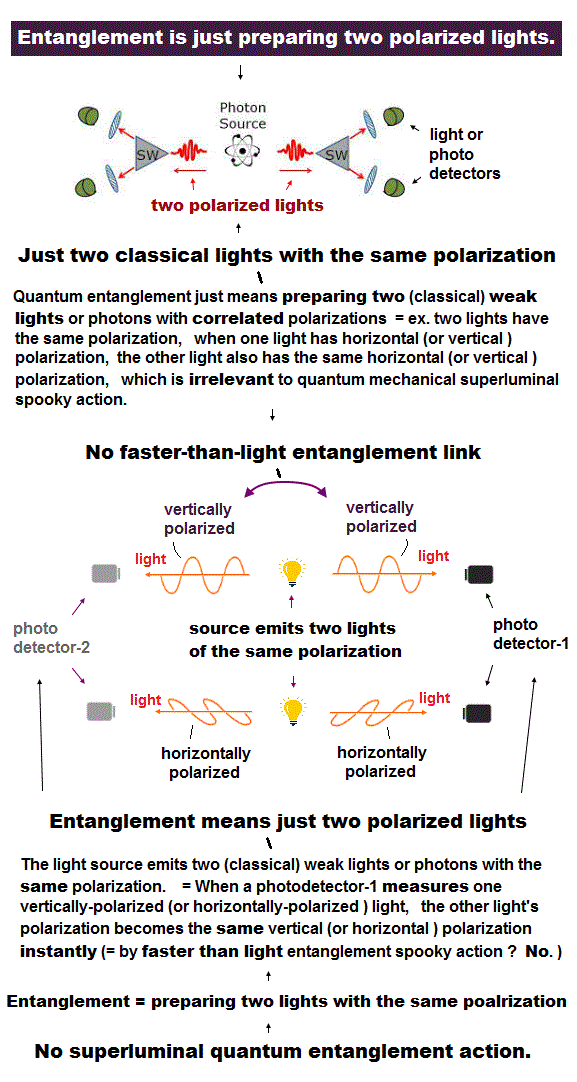The media and academia repeatedly spread fake news that quantum entanglement or spooky action indicates a superluminal link denying any realism, spawning many quantum mechanical fantasies such as parallel worlds.

This quantum entanglement or teleportation (= equal to entanglement ) cannot send any real information or do any work such as communication ( this 4th-paragraph,  this 10th-paragraph ), so the entanglement is a meaningless useless concept except for this.

Actually, the (illusory) quantum entanglement has No practical application, as seen in the still-useless (= forever ) quantum computer, quantum information or quantum internet (= impractical forever ).

If the misleading claim that the spooky entanglement could really send information faster than light is true, it clearly contradicts and disproves the current mainstream science where Einstein relativity prohibits any superluminal information ( this 6-7th-paragraphs,  this p.4-6 ).

Then, why did physicists mistakingly start to say such an illusory and contradictory superlumial entanglement link may actually happen ( this 5-8th paragraphs ) ?

In fact, this illusory quantum entanglement is just about "measurement of some states (= Not the act of sending real information,  this p.11-introduction )".

Physicists prepared two lights (or photons ) with some particular polarizations (= on this page, we use the case of two lights with the same parallel vertical-vertical = VV or horizontal-horizonal = HH polarization ), and they call these polarized lights "entanglement", which has nothing to do with the faster-than-light action.

When they measure one light (or photon ) as horizontally-polarized (= H ), this measurement instantly (= superluminal link ? No way ! ) determines the other light's polarization as horizontal ( this p.2-3rd paragraph,  this p.4,  this 2nd-last paragraph,  this 2-6th paragraphs ).  ← That's all. Entanglement is Neither a superluminal link nor sending actual information ( this last-paragraph,  this 2nd-last paragraph,   ).

When they artificially prepare two light waves (= or fictitious photons ) to have the perpendicular (= orthogonal ) polarizations in advance, if one light (= or photon ) is measured as horizontally-polarized (= H ), it instantly determines (= superluminal entanglement action ? No ! ) that the other photon is vertically(= V )-polarized ( this 3rd-paragraph,  this 2nd-paragraph,  this 3rd-paragraph,  this 3rd-paragraph,  this 2-6th paragraphs ).

In fact, the illusory superluminal quantum entanglement is caused by intentionally misinterpreting the ordinary classical polarized light wave as a fictional indivisible photon particle.

We explain this misinterpretation of entanglement and Bell theorem or Bell CHSH (= Clauser, Horne, Shimony and Holt ) inequality violation based on the illusory photon pair here.

## Quantum entanglement needs fantasy many-world interpretation to avoid violating Einstein relativity.

(Fig.15') Superluminal nonlocal quantun entanglement vs. Quantum parallel-world theory with hidden variable compatible with Einstein relativity forbidding superluminal link.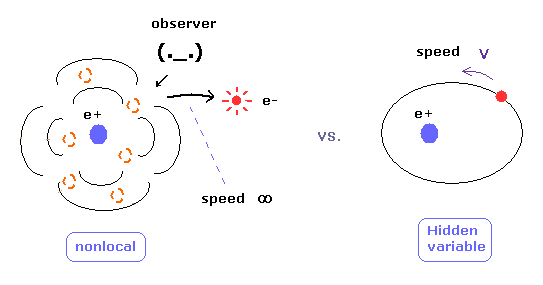If the (useless) quantum entanglement or seeming superluminal spooky action is true, the quantum mechanics needs the fictional parallel worlds or many-world interpretation to avoid the clash with another mainstream science = Einstein relativity forbidding any superluminal actions ( this 5th-paragraph ).

↑ This is why the unrealistic parallel-world theory is accepted as the mainstream interpretation of quantum mechanics, as shown in the parallel-world quantum computers ( this 4th-paragraph ) which are unrealized forever.

↑ But even if physicists tend to choose (fantasy) many-world intepretation as the current mainstream physics, after all, the many-world interpretation also needs some superluminal spooky signal to determine which polarization or which world the distant entangled photon instantly chooses to explain the quantum superluminal entanglement, hence, it cannot avoid contradicting another mainstream physics = Einstein relativity ( this p.1 ).

The (illusory) superluminal entanglement action is called "nonlocal", and the realistic action slower than light is called "local" or hidden variable theory.

## Misinterpretation of classical polarized light wave as illusory photons causes the illusory superluminal entanglement idea.

### [ Unphysical quntum mechanics claims that two entangled photons with the correlated, arbitrary polarizations existing in parallel worlds instantly collapse into a single photon's pair, when they pass the polarizing filters by superluminal entanglement ! ]

(Fig.16) The moment light (or photon )-A is measured as vertically-polarized, the photon-B also becomes vertically-polarized through (illusory) superluminal or instantaneous entanglement action ?  ← This is wrong.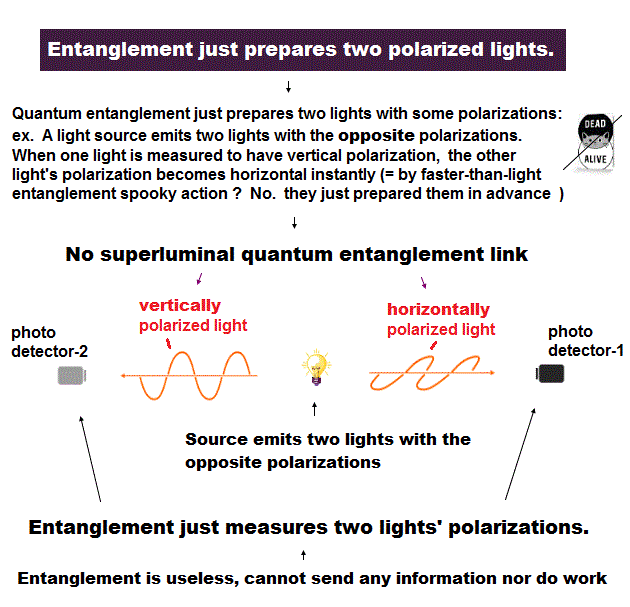In 1982, Alain Aspect showed that in the test of Bell entanglement theory, the Bell inequality (CHSH) which should satisfy local realism was violated using the alleged entangled photons, and claimed that it indicated the existence of the faster-than-light entanglement link between two photons.

But in fact, this false contradictory claim of the faster-than-light entanglement is caused by intentionally mistaking the ordinary divisible classical polarized light waves for the illusory indivisible photon particles.  = illusory photons are dotted circles with fictitious polarizing arrows ( photon's polarizations of 0o, 90o and an arbitrary angle θ are shown in the uppre figure ).

### [ Quantum ( illusory superluminal ) entanglement experiments use the ordinary classical divisible electromagnetic wave, Not a fictitious indivisible quantum photon particle, so the superluminal entanglement link is illusion. ]

In (illusory) superluminal quantum entanglement experiments, it is said that physicists used a pair of two polarized photons (= particle ? ), which were emitted from some special crystal or excited atoms.

Here we use the case where these two "alleged-entangled" photons are prepared to have the same parallel polarizations, when one photon A has vertical polarization (= V ), the other photon B also has vertical polarization,  or when one photon A has horizontal polarization (= H ), the other photon B also has horizontal polarization.

Whether each photon has vertical or horizontal polarization is unknown and indistinguishable, until the measurement (= this condition is necessary for quantum entanglement,  this 3rd-paragraph,  this 3rd-paragraph ).

And when an observer measures one photon A as vertically-polarized, it instantly determines (= superluminal entanglement action !? No ! ) the distant other photon B as vertically-polarized ( this p.2-3,  this p.4,  this 2-6th paragraphs,  this 2nd-last paragraph ), because these two photons A and B are prepared to have the same polarization in advance.  ← This is the current ridiculous superluminal quantum entanglement logic, though physicists never show any detailed physical mechanism of this occult entanglement link.  ← Science stops progressing due to fantasy quantum mechanics forever.

In fact, physicists use the ordinary classical electromagnetic wave and Maxwell electromagnetism as a fictitious quantum photon in entanglement experiments, so the occult superluminal quantum entanglement is impossible.

When physicists generate a pair of two alleged entangled polarized photons, they try to split one single (pump) photon into two daughter photons (= idler, signal ) with lower energy or frequencies by the method called "parametric down conversion" that just passes an incident photon (= just classical light wave or laser ) through the special crystals ( this p.4,  this p.2-lower ).  ← No magical superluminal entanglement

↑ First of all, it is impossible for a ball-like rigid photon particle (= if it existed ) to have the wave-like polarization or wave frequency, which is clearly the wave nature, Not a particle.

And the idea that an indivisible photon particle can split into two daughter entanged photons is self-contradictory and wrong.

In this spontaneous parametric down-conversion allegedly splitting an incident (indivisible) photon particle into two daughter entangled photons (= contradict ! ), they use the ordinary classical Maxwell electromagnetism where the incident divisible classical light wave's electric fields oscillate the atoms inside the crystal, and this atomic oscillation by the light electric field emits two electromagnetic waves, which process has nothing to do with a quantum indivisible photon (= but they falsely treat these newly-generated classical divisible electromagnetic light waves as indivisible fictitious quantum entangled photons,  this p.5-6,  this p.3-6,  this p.19 ).

In the type-1 down-conversion, an incident photon passing through the nonlinear BBO crystal paradoxically splits into two daughter entangled photons with the same polarizations = when one photon has the vertical (= V ) polarization, the other photon also has the vertical (= V ) polarization (= VV ),
Or when one photon has the horizontal polarization (= H ), the other photon also has the horizontal polarization (= HH,  this p.5 ).

↑ Unphysical quantum mechanics claims that these two photons are in the fictional superposition states (= a dead and alive cat state ) where their different-combination polarization states of the horizontal-horizontal polarization (= HH ) and vertical-vertical (= VV ) polarization should exist simultaneously ( this p.2-left,  this p.5 ) using fictional parallel worlds ( this 3rd-paragraph ).

↑ Each single photon haivng the same chance of vertical and horizontal polarizations means each photon is unpolarized ( this p.5-last-paragraph,  this p.4-(c) ) or having random polarizations (= two entangled photons have many different parallel worlds of the same but arbitrary polarizations simultaneously,  this p.9,  this p.7-8,  this p.2-right ).

Because a (fictitious) photon is said to obey the ordinary Malus law (= light intensity passing the filter at an angle of θ becomes Icos2θ ), and the chance that a photon with vertical polarization passes the polarizing filter at an arbitrary angle θ is cos2θ, the chance that a photon with horizontal polarization passes the same filter is sin2θ, hence, the total chance is cos2θ + sin2θ = 1 = always the same chance 1 in any arbitrary polarization angle θ.

Quantum mechanics illogically claims when a photon-A passes the polarizing filter A, and the photon-A's polarization becomes the same as the filter-A, the distant photon-B's polarization instantly (= superluminal or instantaneous entanglement !? ) changes into the same polarization as the distant polarizing filter-A's axis, or the photon-B with the same polarization as the distant filter A in one of different parallel worlds is conveniently chosen by superluminal telepathy or entanglement as one left photon's measurable polarzation state ( this p.2-lower ).

↑ In this case, when photon-A passes the filter-A, the chance of photon-B passing the filter-B becomes cos2θ when θ is the angle difference between the filter-A and B's axes, which violates Bell inequality, but these dubious expermerimental results can be naturally explained by the ordinary classical light wave even without fantasy superluminal entanglement.

In the realistic interpretation using the ordinary classical light wave, an incident (divisible) light wave splits into two daughter light waves with the same arbitrary polarization (= when one light has the polarization with the angle θ, the other light also has the same polarization angle θ ), and the light intensity passing the polarizing filter is cos2θ which is related to the chance of the passing light intensity exceeding the detection threshold of the photodetector ( this p.2-7 ).

Aspect used the excited calcium atoms moving in random directions as a source of entangled photons (= just classical light wave,  this p.20 ), whose polarization points in the random directions satisfying one condition that these emitted paired photons (= or two lights ) have the polarizations parallel to each other.

In type-2 down-conversion case, two entangled photons have the orthogonal polarizations = when one photon has vertical (or horizontal ) polarization, the other photon always has the different horizontal (or vertical ) polarization = each photon is measured to have an arbitrary polarization orthogonal to the other photon's polarization ( this p.7-2nd-paragraph,  this p.39-top ).

↑ Also in this case, the realistic light wave can match the experimental results even without the unrealistic superluminal entanglement.

When an incident light splits into two daughter lights: one light has vertical polarization and the other light has horizontal polarization, these two light with the orthogonal polarizations can be changed into circularly-polarized light by the wave phase shift (= or electrons inside crystals are oscillated in the orthogonal + circular directions designated by the crystal orientation by the orthogonal electric fields of the incident light, emitting two daughter lights with half the original frequency ), which means these two light waves can have the arbitrary polarizations orthogonal to each other ( this p.2 ).

↑ Or, when the incident light goes through the special crystal causing the type-2 down-convsersion, this light oscillates some atoms inside the crystals in two othogonal directions (= the emitted light's polarization direction is arbitrary depending on the directions and arrangements of these atoms in slightly-different places inside the crystal ) and lets them emit two daughter lights with mutually-orthogonal (= and arbitrary) polarizations.

## Probability of a (fictional) polarized photon (= light ) passing the polarizer is wrong in quantum entanglement experiments.

(Fig.17) Probability of a ( illusory unseen ) photon passing a polarizing filter obeys the same Malus law of classical light wave's intensity (= I ) passing the polarizing filter with the probability cos2θ where θ is the angle between the polarization of classical light (or a fictitious photon ) and the polarizing filter's axis ?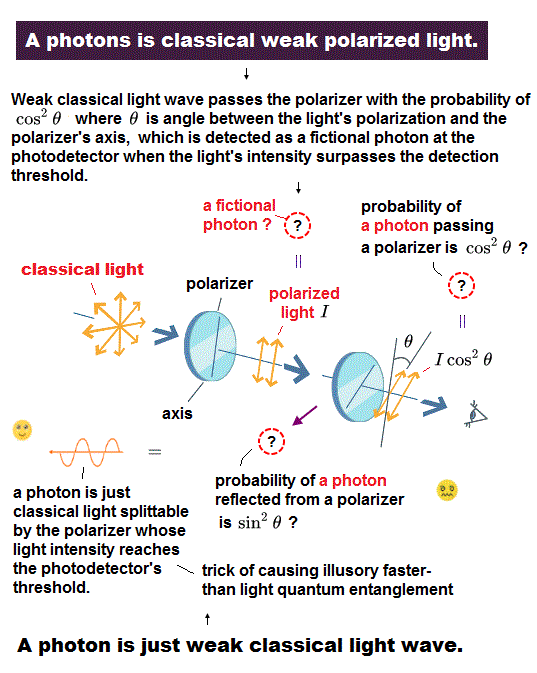According to the classical Malus' law, when the angle between the polarization axis of the electromagnetic light wave and the polarizing filter (= polarizer ) is θ, the classical divisible light splits into two weaker light waves at the polarizer where the light intensity passing the polarizer is I cos2θ and the reflected light intensity is I sin2θ (= of course, this rough probability estimation is different from the fact, considering the light loss ).

The point is this classical Malus' law is valid only in the classical divisible light wave, Not valid in the illusory indivisible photon particle.

But physicists tries to falsely apply this classical Malus' law also to the unseen illusory photon particle, which is why the unrealistic superluminal quantum entanglement idea is created ( this p.2-last,  this 9-11th paragraphs,  this p.5,  this p.5-2nd-last paragraph ).

## Probabilities of (illusory) photon's passing or reflecting at polarizing filter ?

(Fig.18) Pass or reflect probability of a single photon at filter is false.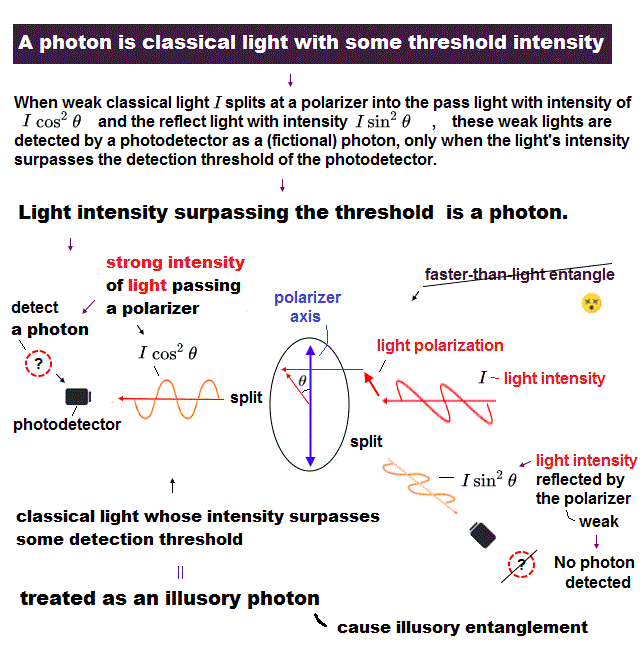Quantum mechanics falsely claims that even a illusory indivisible photon particle (= quantum particle of light ? ) satisfies the classical Malus' law ( in a little modified way, though, because the original Malus law is based on the divided classical light wave at the polarizer ).

They insist when a (unseen) photon particle with red arrow as the fictitious polarization hits the polarizing filter, this unseen photon can pass through this polarizer with the probability of cos2α where α is the angle between polarizer's axis and the photon's polarization ( this p.5,  this-(4),  this p,5-(11) ) without dividing each photon particle at each polarizer, which is different from the original Malus law.

And they insist the photon reflects off the polarizer with the probability of sin2α (= cos2α + sin2α = 1 ?  this p.7 ) without dividing each photon particle.

The problem is that the single photon detector cannot detect the very weak fragile photon (= just weak classical light wave ) with 100% detection efficiency due to the frequently lost photons and false dark count.

Usually, the photon detection probability or efficiency is far lower than 100% ( this p.8-9 ).  The coincidence detection rate of two entangled photons is less than 20% ( this p.3-4, Fig.2,  this p.3-Table.I,  this Fig.2, Table I )

↑ This low photon (coincidence) detection probability at single-photon detectors causes the illusion such as faster-than-light spooky entanglement, which is called "detection loophole ( this p.3-2nd-paragraph,  this p.4-left-lower )".

In order to eliminate the possibility of classical light wave, they need to achieve not only the high detection efficiency of each weak photon but also the extremely high coincidence detection rate of two paired photons, which is impossible in the photon entanglement experiments.

## Fictitious classical photon's case (= Not realistic classical light wave ) -- Photon's passing probability = photon A's probability × photon B's probability ?

(Fig.19) The probability of both ( fictitious classical ) photons passing their polarizing filters ?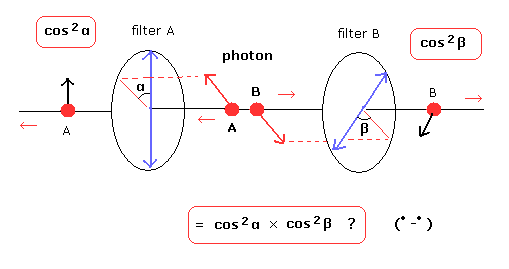The fatal flaw of the (illusory) quantum entanglement is physicists falsely try to compare the quantum photon entanglement experiments with the unreal classical indivisible photon particle (= Not real classical divisible light wave ), though such an indivisible classical photon particle does Not exist.

On this page, we use the case where two paired photons whose polarizations are parallel to each other are emitted in the opposite directions, and the photon A hits the polarizing filter A and the photon B hits the polarizing filter B.
Each photon passes or reflects from each filter it hits depending on the angle (= α or β in the upper figure ) between the photon's polarization and polarizing filter's axis.

The angle of each polarizing filter's axis can be freely changed in advance.

When the angle between the (fictitious classical) photon A's polarization and the polarizing filter A is α, the probability that the photon A passes through the filter A is cos2 α ( ← (E cos α)2 ). (The reflection is sin2 α) using the classical Malus law.

When the angle between the photon B's polarization and the filter B's axis is β, the probability of the photon's passing the polarizer is cos2 β, and the reflection is sin2 β.

In this case, for example, the probability that both these (fictitious classical) photon particles pass their filters is ( cos2 α × cos2 β ).

But this case satisfies the Bell inequality (= hence, no entanglement ) and is different from the experimental results, so physicists claim that (illusory) superluminal quantum photon's entanglement link is necessary, instead of adopting the real divisible classical light wave.

## (Illusory) superluminal "spooky" link or entanglement actually happens ?

(Fig.20) Experimental results. ( = proving fantasy faster-than-light entanglement ? ) = chance of both photons passing (and both photons reflecting from ) their filters is cos2θ where θ is the axis angle difference between two polarizing filters A and B.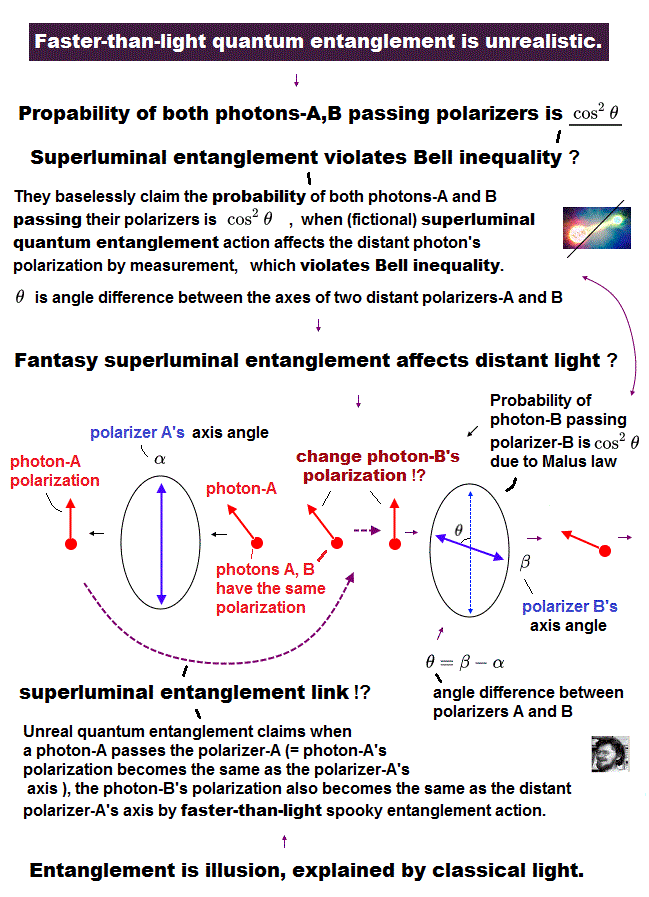The actual probability of detecting two photons (= lights ) passing each polarzing filter is different from Fig.19 of two fictitious classical photons.

The probability that both of the two photons pass each filter is about 1/2 cos2 (β - α) ( not cos2 α × cos2 β,   this p.4,  this p.33,  this p.2 ) !

Here, θ = (β - α) means the angle difference between the axes of two polarizing filters A and B.
The number of the coincidence count of two photons passing their filters seems to roughly obey the curve of cos2θ ( this p.4-Fig.3,  this p.3-Fig.2 )

So physicists (falsely) claim that the quantum faster-than-light entanglement is real, violating the Bell inequality in some filter's angle θ.  ← Nonsense.

Because if the probability of two (fictitious) photon particles A and B passing their polarizing filters A and B (= angle difference between two polarizers' axes is θ ) is cos2θ, the following unrealistic interpretation is needed, when you use the indivisible photon particles.

When the photon A (whose polarization angle is different from the filter A axis by arbitrary α ) passes the filter A (= the photon A's polarization becomes parallel to the filter A's axis, after the photon A passes the filter-A ), the other photon B polarization instantly (= by superluminal entanglement !? ) changes into the same angle as the distant filter A's axis (= same as the passing photon A's polarization ), then the pobability of the entangled photon's B passing the filter B is cos2θ (= instead of cos2β ) where θ is angle difference between two distant polarizers' axes due to Malus law ( this p.3-1st-paragraph,  this p.2-right,  this p.3-4 ).

The chance of the photon's A passing the polarizing filter A is supposed to be 50% due to the random light polarization setting (= under the condition that polarizations of two photons A and B are parallel to each other ), so the total probability of both photons A and B passing each filter becomes 1/2cos2θ = 1/2cos2(β-α); = 1/2 ( θ = 0,  this p.41,  this p.2-3 ).

In the same way, when two polarizers's axes point in the same direction ( θ = 0 ), the probabilities of two photons A and B are reflected by each polarizer is also 1/2cos2θ = 1/2, because they ridiculously claim that when photon A is reflected by the filter A (= the reflected photon A's polarization becomes perpendicular to the filter A's axis ), the photon b's polarization instantly (= superluminal entanglement action !? ) becomes perpendicular to the distant filter A's axis (= photon B polarization instantly becomes the same as the distant reflected photon A's polarization ) by the (illusory) superluminal entanglement action ( this p.9,  this p.2-3 ).  ← Impossible !

The total probability of two photons A and B giving the same results (= pass-pass + reflect-reflect ) equals 1/2+1/2 = 1 = cos2θ ( θ = 0 ), which probabilities are allegedly given by the quantum superluminal entanglement theory connecting two distant particles with supernatural occult power.

But as I said, this ridiculous claim of superluminal entanglement is the result of the misinterpretation of the realistic divisible classical light wave as a fictitious indivisible photon particle.

## The instant one photon passes ( or reflects from ) its filter, another distant photon's polarization changes by the (illusory) superluminal instantaneous entanglement action !?  ← Nonsense.

(Fig.21) Quantum entanglement is caused by faster-than-light "spooky" link ?  ← Quantum mechanics is just pseudo-science lacking reality.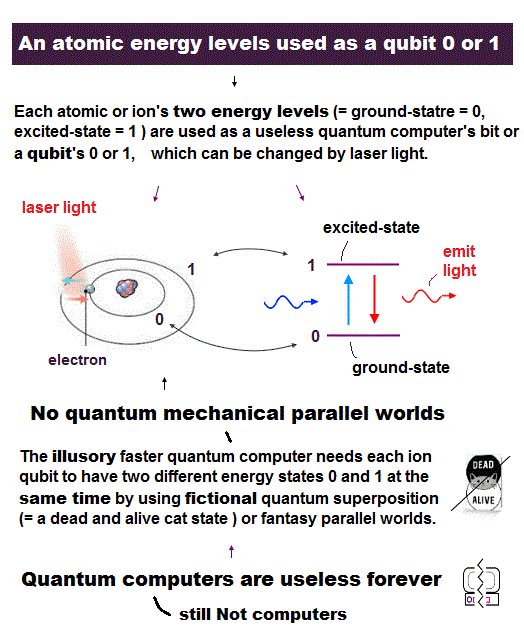Physicists unrealistically claim that when the photon A passes (or reflects from ) the polarization filter A, the photon A's polarization becomes parallel (or vertical ) to the filter A's axis, and it instantly makes the distant photon's B polarization the same as the photon's A polarization (= photons A,B polarizations instantly become the same parallel even after they pass their different filters far away from each other ) by the (illusory) fanster-than-light entanglement action.

As I said, if this fantasy superluminal quantum entanglement is right, the current mainstream science of Einstein relativity forbidding any faster-than-light transmission is wrong.

So the current mainstream physics based on (fantasy) quantum mechanics and Einstein relativity is self-contradictory and wrong.

## Fictitious "rigid" particle called "photon" is the cause of "unreal" entanglement spooky link.

(Fig.21') Unreal photon particle is not divided ← Unreal superluminal entanglement occurs.  Realistic light wave is divided. ← No spooky link needed.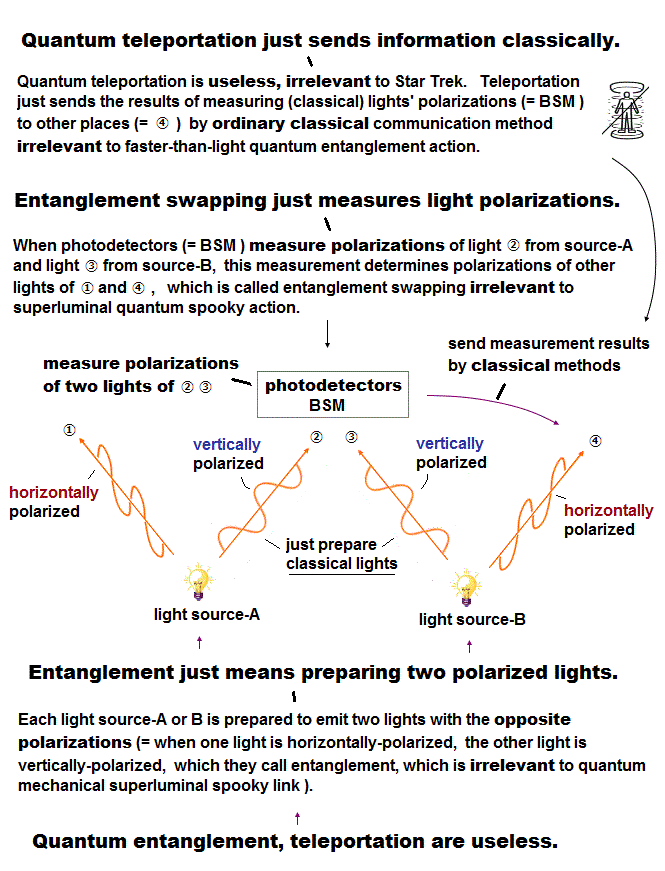Illusory idea of superluminal entanglement is caused by physicists intentionally mistaking the realistic divisible classical light wave for an illusory indivisible photon rigid particle.

The indivisible photon particle is illusion, as seen in extremely lower photon detection efficiency and coincidence detection rate of two paired (entangled) photons (= a very weak photon easily splits into weaker light waves which can no longer be detected at the single photon detector, and disappears ).

If the photon is a realistic light wave, the passing photon A can be detected only when the polarized angle of the photon A is near the angle of the polarizing filter A's axis due to the detecting threshold ( this p.2-7 ).

For example, when the angle α between the photon A's polarization and the filter A's axis is about π/4 or 45o (= middle point), the photon A = light wave A is divided into the pass and reflect sides equally at the filter (= light intensities passing and reflecting from the filter A become the same cos2π/4 = cos245o = 1/2 of the original light intensity due to Malus law ) and detected on neither the pass nor reflect sides of the filter.

Because both the pass and reflect light intensities (= 1/2 × I ) become equally divided and weaker to the half of the original light intensity (= I ), which cannot reach the detection threshold (= Fig.21'') of a single-photon detector.

For example, we can suppose the single photon detector can detect the weak (split) light as a (fictitious) photon, only when its light intensity exceeds the photon detection threshold of 0.7 × I (= the photon detector's threshold can be artificially adjusted ) in which case, the equally-divided photon or light intensity = 0.5 × I cannot be detected by the single-photon detector as a (fictitious) photon.

When we cannot detect two prepared (entangled polarized) photons A and B at the same time (= coincident detection ), this case is NOT counted as a entanglement result (= entanglement experiments see only the cases of detecting two photons A and B simultaneously, which two photon coincidence detection rates are extremely low,  this p.4-left-lower ).

## When classical lights are divided into weaker light → they cannot reach the detection threshold of a single-photon detector.  ← Trick of illusory superluminal entanglement

(Fig.21'') Wave is equally divided = not reach detection threshold.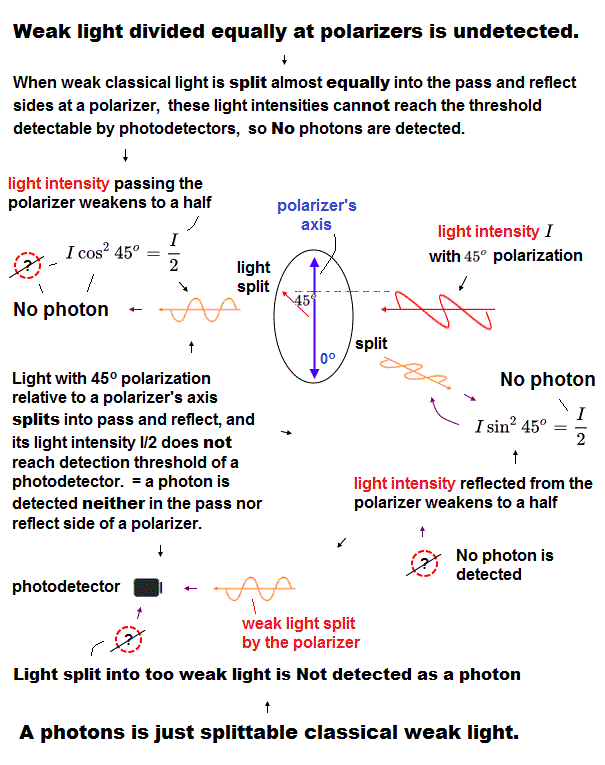When we detect two photons A,B at the same time at each single-photon detector, it is possible that those two photons (= just weak classical lights ) originally have the polarization angle near the polarizing filters they hit, in which case, most of those light waves whose polarization is near the filter's axis can pass each filter enough to exceed the photon's detection threshold.

When the angle between the photon (= or light ) polarization and the polarizing filter's axis is about 45o or π/4 as shown in the upper figure, the photon (= or light wave ) intensity is almost equally divided into the pass and reflect sides of the filter (= the pass or reflect light intensity weakens to almost half of the original light intensity due to Malus law, Icos2π/4 = 1/2 × I ), and this equally-divided photon (= light wave ) cannot be detected as a photon on either side of the polarizing filter due to Not reaching the detection threshold of the photon detector ( this p.4,6 ).

It is certain that there is some "minimum threshold light amplitude or intensity" required to excite a single electron (= photoelectron ) from the photodetector (= light with zero intensity excites no electron, → there's some minimum light intensity which starts to excite the target electron at the photodetector ).

This means that the "wave nature" of the divisible light causes the false idea that the (illusory) superluminal quantum entanglement is occurring.

On this page we use the two (entangled) photons (= light waves ) whose polarizations are parallel to each other (= which direction both these photons's polarizations point is random and uncertain until the measurement ).

If you want to use a pair of the vertically-polarized and horizontally-polarized photons as entangled particles, change cos2 θ into sin2 θ, which meanings are the same as far as they satisfy Malus's law.

## Classical light wave can perfectly explain Bell inequality violation, so the fantasy superluminal entanglement is unnecessary.

(Fig.22) Directions (= axes ) of two distant polarizing filters A and B are the same or parallel to each other. ( θ = 0 degrees )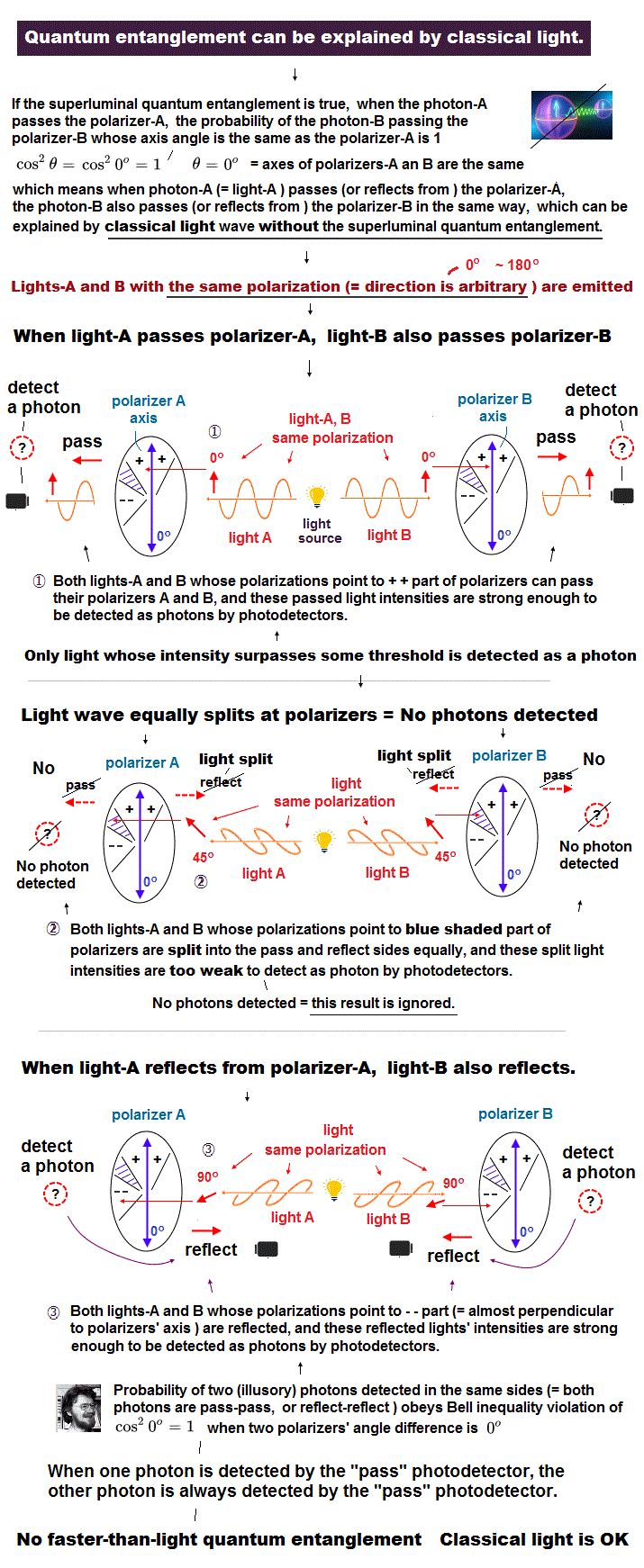We arrange for these two photons or light waves to have the same parallel polarizations (= vertical-vertical, horizontal-horizontal, 45o-45o, 80o-80o ... polarizations. Which direction these two lights point is unknown until the mesurement like "unpolarized" light,  this 3rd-paragraph,  this p.4-(c), ).

The light polarization directions are uncertain and randomly changed (= like unpolarized light,  this p.5-last-paragraph ) under the condition of two light wave polarizations always parallel to each other (= or the directions of two polarizing filters' axes are randomly changed under the condition of the angle difference between two polarizing filters' axes is always the constant.  In the upper Fig.22 case, two polarizing filters' axis angles are the same parallel ).

This figure shows the case in which the angles ( = the directions of the arrows) of the axes of two polarizing filters A and B are the same ( = 0 degree).

So in this case, when photon A passes through filter A, photon B always passes through filter B, ( = cos2 0 = 1 ), which detection probability agrees with the experimental results (= of illusory superluminal entanglement according to quantum physicists,  this 6th-paragraph,  this p.53 ) even without using the superluminal entanglement action, when we replace fictitious photons by real divisible classical light wave.

So the fictional superluminal quantum entanglement is unnecessary.

The polarization directions of two photons are random, except for one condition that these two light polarizations are always parallel to each other.

When the light polarizations of these two photons point in the direction of ( ++ ) of the upper figure, most light intensities of both these photons (= divisible light wave ) A and B can pass through their polarization filters, and can be detected as the passing photons by exceeding the detection threshold of the single-photon detector ( this p.7-2nd-paragraph ).

When these photon polarizations point in the direction of the blue region of the polarization filters, neither photon A nor B can be detected, because the photon (= light ) is almost equally divided in the pass and reflect sides of the polarizing filter, and their light intensities cannot exceed the photon detection threshold of photodetector ( this p.2-7 ).

When the light polarizations of these two photons point in the direction of ( -- ) of the upper figure, most light intensities of both these photons (= divisible light wave ) A and B are reflected by their polazation filters, and can be detected as the reflected light (= photon ) by exceeding the detection threshold of the photodetector.

↑ As shown here, we can perfectly explain the experimental results (= Bell inequality violation ) using the ordinary divisible classical light wave even without relying on the contradictory superluminal entanglement action.

## Detection "threshold" at photodetector is a key player to cause the illusory superluminal quantum entanglement.

(Fig.23) Most of both the divisible light waves A and B pass their polarizing filters ( ++ ), then, their passing light intensities remain strong enough to be detected as (fictitious) photons at the photodetector.  ← Trick of fictional superluminal entanglement !When the polarization direction of the photons A ( this photon A's polarization direction is the same as the photon B, because these two photons' polarizations are prepared to be parallel to each other ) points toward ( ++ ) of Fig.22, both the photons A and B are likely to pass each filter, and are detected as "photon particles", because these light (intensity) can pass these filters enough to be detected at the photodetector (= Fig.23 ).

The pass light intensity is I cos2α ( or I cos2β ) due to Malus law.
When the light polarization points to (++), this angle α (or β ) between the light polarization and the filter's axis is near 0 degrees, so the light intensity passing the polarizer is strong enough to exceed the detection threshold and this passing light wave with enough intensity is detected at the single-photon detector as a (fictitious) photon.

## When two polarizing filter directions are the same ( θ = 0 ), both photons (= lights ) always give the same results (= chance of both photons passing and reflecting is con2θ = 1 ).

(Fig.24) Both classical light waves are split almost equally = not detected at a single-photon detector due to their equally-split light intensities not reaching the detection threshold.But when the polarization direction of the photon A( = the same as photon B polarization ) points toward the blue part of Fig.22, the transmitted (or reflected) light intensities are below detection threshold of a single-photon detector, because these lights are divided almost equally into pass and reflect sides of the polarization filters obeying the classical Malus' law.

For example, the pass light intensity is I cos2α and the reflect intensity is I sin2α.
When this angle α is near 45 degrees (= π/4 = blue part of Fig.22 ), both pass and reflect light intensities become 1/2 × I. ( 1/2 = cos2 π/4 = sin2 π/4. See also Eq.21''. )

In this case, both pass and reflect light intensities become weaker by being equally split into almost half of the original light intensity.
So neither photon A nor B can be detected as a "photon particle" by the detector (this case is not used or counted for the entanglement or calculating the detection efficiency, because both photons are undetectable ).

## There is NO spooky entanglement link. It's caused by photon's detection threshold and the divisible classical light wave.

(Fig.24') Both light waves (= intensities ) reflect ( - - ) from polarizers enough to be detected at the single photon detectors as (fictitious) photons.And when the polarization direction of the photon points toward the ( -- ) of Fig.22, both the photons A and B are reflected by the polarizing filters enough to be detected as "photon particles" due to their reflected light intensities exceeding the detection threshold.

For example, the reflect light intensity is I sin2α. when this angle α is near 90 degrees (= (--) part of Fig.22 ), the reflect light intensity becomes strong enough.
So in this case, the "illusion" of the entanglement of these paired photons are occurring (= if we consider the fictitious photons to be the realistic divisible light wave, this illusory superluminal entanglement link is unnecessary ).

In Fig.22 case where the axes of two polarizing filters A and B point in the same direction (= θ = α - β = 0 ), the probabiliy of both the photon A and B giving the same results (= both photons passing their polarizers + both photons are reflected by their polarizers ) become cos2θ = 1, as predicted by (illusory) photon's entanglement theory.

## Illusory quantum entanglement is caused by comparison with (fictitious) indivisible classical photons.

(Fig.I) There are No such things as indivisible classical (or quantum ) photons.As shown in the upper Fig.I, physicists falsely assume that the (fictitious) indivisible classical photons pass or reflect off each polarizer obeying Malus' law, though Malus's law originally applies only to the realistic classical light wave Not a photon particle.

In this fictitiously indivisible classical photon case, when photon A passes the filter A, the photon B passes or reflects, even when the axis angle difference between the polarizing filter A and B is zero ( θ = 0 ).

So the results of photon A and photon B are NOT always the same even in θ = 0, which disagrees with the experimentally observed probability of cos2θ

As a result, the illusory superluminal quantum entanglement action is caused by the false assumption of the illusory quantum or classical indivisible photon particles.  ← Realistic classical light wave can perfectly explain the experimental results without needing the unrealistic entanglement.

This is the trick of the illusory superluminal quantum entanglement.

## Entangled photon particle can be replaced by the realistic divisible classical light wave combined with the detection threshold at the single photon detector.

(Fig.II) Illusory entangled photon particles can be replaced by realistic divisible classical light wave.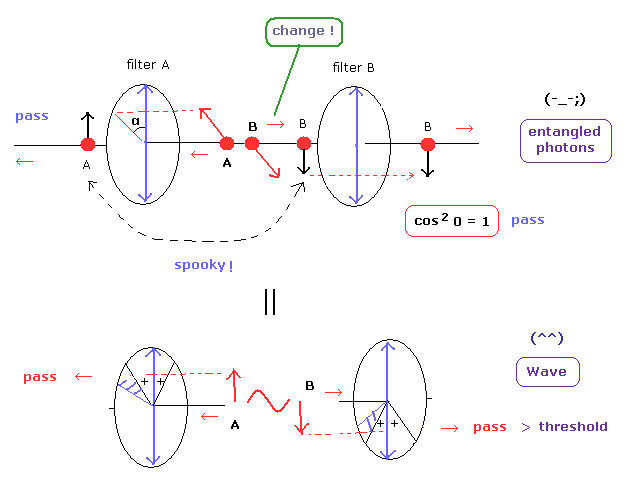In entangled photon pair, when the photon A passes the filter A, the photon B always passes the filter B due to the unrealistic superluminal spooky link which allegedly can change the distant photon's polarization instantly and superluminally with unknown supernatural power.

Instead of accepting this unrealistic ridiculous entanglement link idea, it is more natural to think a photon is just classical divisible electromagnetic waves detected as "photon", only when the classical light intensity after splitting exceeds the detection threshold of a single-photon detector.

## The case when the angle between two polarizing filters is 90 degree can be also perfectly explained by classical light wave.  ← Quantum entanglement is unneeded.

(Fig.25) Angle between polarizing filters A and B is θ = 90 degrees.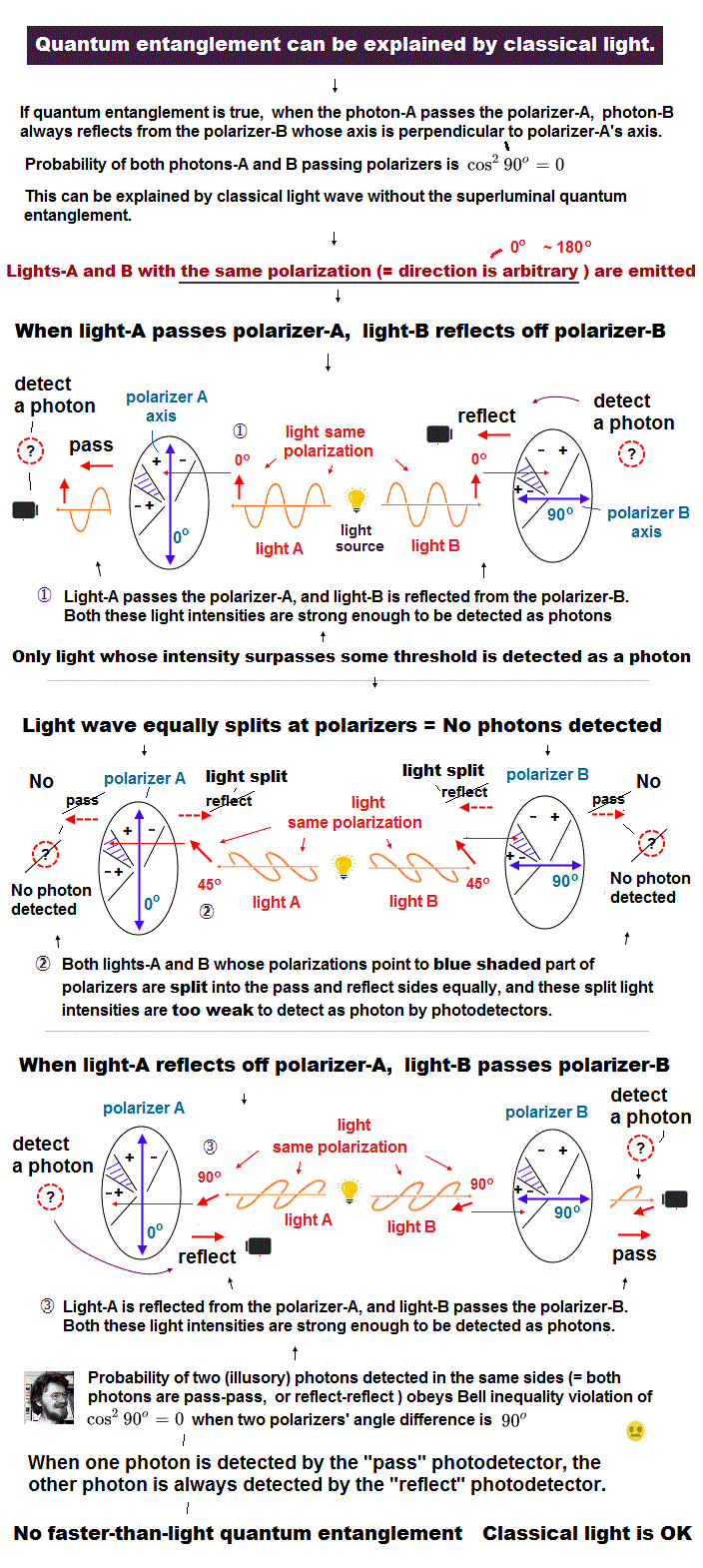Next, this figure shows the case in which the axis angle difference between the polarizing filters A and B is 90 degrees (= two polarizers' axes are perpendicular to each other ).

The photon A and B polarizations are always the same, parallel to each other, as I said.

In this case, when the photon A passes through the filter A, the photon B is always reflected by the filter B ( = cos2 90 = 0 ) according to the entanglement theory.

When the polarization of the photon A ( = the same direction as photon B's polarization ) points toward (+ -), the photon A passes the filter A, and the photon B is reflected by the filter B whose axis is perpendicular to the filter A (and both photons or lights can be detected as "photons" exceeding the detection threshold due to their light intensities not equally divided at the filter ).

## Two photons always give different results (= one photon is "pass", another is "reflect" ) when the axis angle difference between two polarizing filters A and B is 90o

(Fig.25') Photon A passes, photon B reflects ( + - ).But the polarization of both photons points to the blue part of this figure (= both photons's polarizations are prepared to be parallel to each other ), neither photon A nor B can be detected as a "photon particle" due to their light intensities equally splitting and not reaching the photon detection threshold.

Because in this case, the divisible classical light waves (= intentionally mistaken for indivisible photons ) are "almost equally" divided into pass and reflect directions at the polarizing filters (= both pass and reflect light intensities weakens to the half of the original light intensities due to cos245o = sin245o = 1/2 ), which fail to reach the detection light intensity threshold of the photon detector.

## Also when the angles between two polarizing filters's axes is 45 degree, the classical light wave can perfectly explain the experimental results without the fictional superluminal entanglement.

(Fig.26) Angle difference between two polarizing filters' axes A and B is θ = 45 degrees.This figure shows the case in which the axis angle difference between two polarizing filters A and B is 45 degrees.
In this case , when the photon A passes through the filter A and is detected, the probability that the photon B passes the filter B is 50 percent ( = cos2 45o = 1/2 ) according to the entanglement theory.

When the polarization of the photon A ( = photon B's polarization parallel to photon A ) points toward ( ++ ), both the photons A and B can pass each polarizing filter and be detected as "(illusory) photon particles" (= both photons or light waves unequally split into the pass and reflect sides, and the pass light intensity is stronger enough to be detected at the single photon detector by exceeding the detection threshold ).

When the photon polarizations point in the direction of ( +- ), most of the photon A (= divisible light wave ) passes the filter A and most of the photon B is reflected by the filter B and can be detected as "photons" by reaching the detection threshold.

So the probability that both the photons A and B pass each filter becomes 50 % (= cos2 45o = 1/2 ) compatible with the experimental results without relying on the unrealistic superluminal entanglement.

## Detection "threshold" of recognizing the weak divisible classical wave as "photon" is trick of illusory superluminal entanglement.

(Fig.26') Photon A pass, reflect, not detected regions.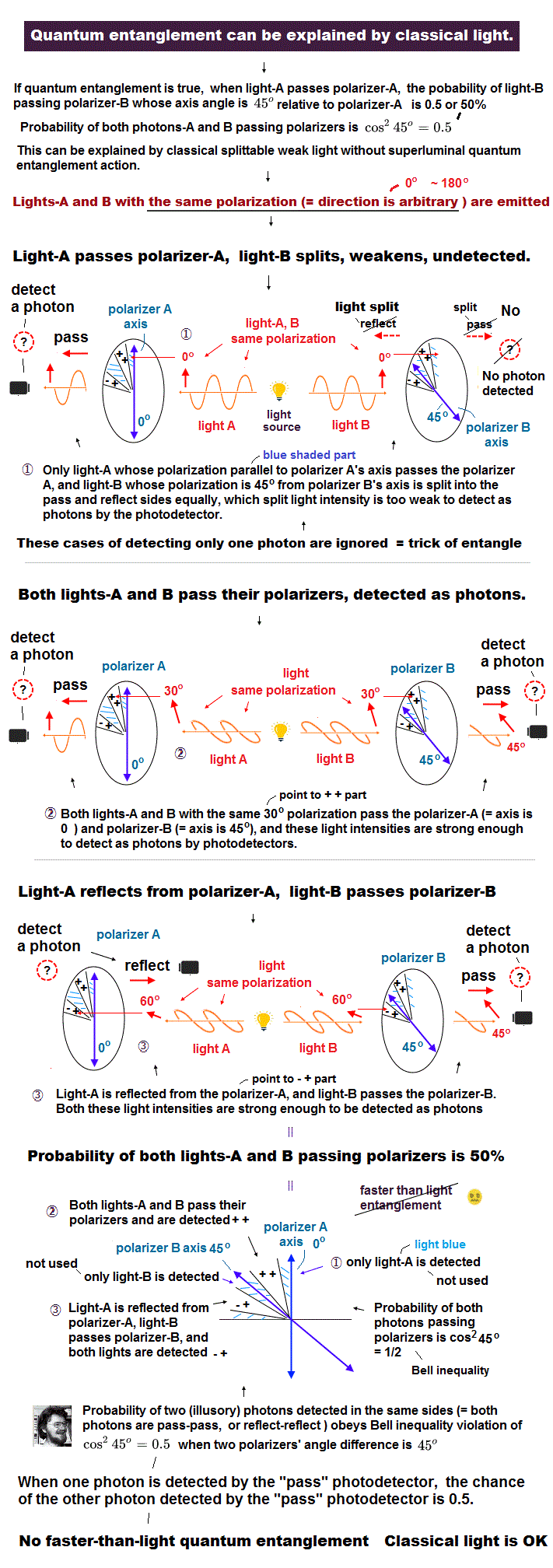When the polarizations of two photons or light waves point in the directions of the blue parts (= both photons A and B are prepared to have the same parallel polarization ), only one of the two photons A and B can be detected as a photon, because one of photon or light wave tends to be almost equally split at the polarizing filter and have weaker transmitted (or reflected ) light intensity than the detection threshold at the photodetector.

If only one of two photons or lights can be detected, this case is not used as the correct experimental result.
Because only when we detect both the two photons at the same time (= coincidence ), we consider these photons to be in the entangled states.
(In the actual Bell test experiment using the photon pairs, the coincidence ( = entangled photon pairs ) detection rate is very low ( < 20% ). So the truly-loophole-free entanglement experiments are impossible.  this p.3,  this p.3-3, Fig.2 )

This example violates the Bell inequality due to the cos2 θ probability. But of course, the unrealistic phenomenon such as the faster-than-light entaglement action is not occurring here.

Here, let's calculate the Bell inequality (CHSH type).
We add one more case in which the axis angle difference between the polarizing filters A and B is 30 degrees.

## Also when the axis angle difference between two polarizing filters A and B is 30o, the classical light wave can perfectly explain the experimental results without the illusory superluminal entanglement.

(Fig.27) Angle difference between two polarizing filters A and B is θ = 30 degrees.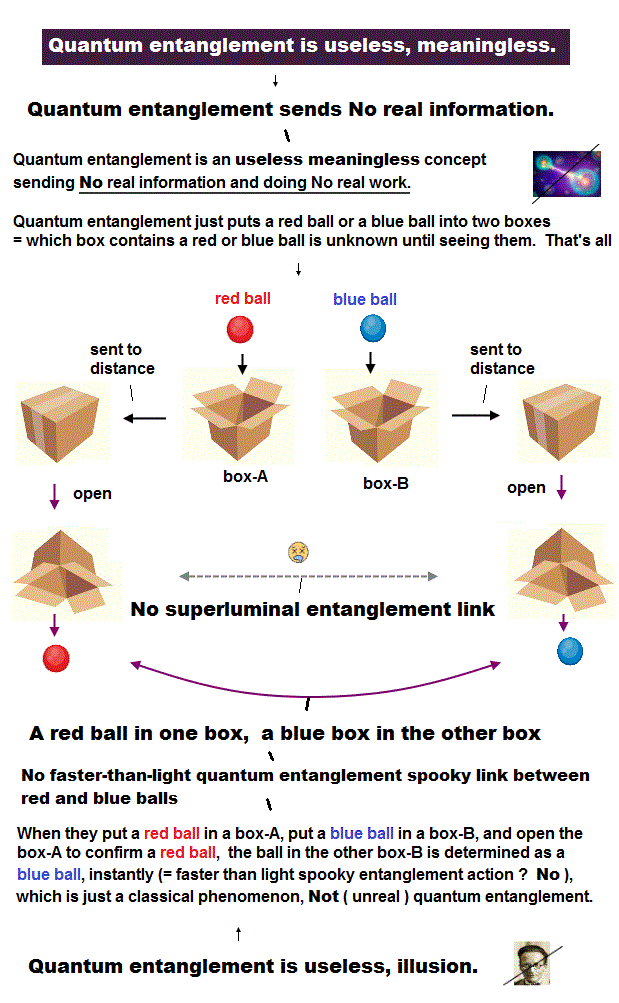We think about the case when the angle difference between two polarization filters A and B axes is 30o.

When two photons or classical light waves with polarizations parallel to each other hit each polarizing filter ( the photon A or light wave A hits the polarizing filter A, and the photon B or light wave B hits the polarizing filter B ), these classical light waves split into the pass and reflect sides depending on the filter's axis.

Only when the light intensity even after splitting at the polarizing filter remains strong enough, it can reach the detection threshold and be detected as "(fictitious) photon" at a single-photon detector.

When the polarizations of two light waves (or photons ) point to the red parts (+ +), both light waves A and B pass each polarizing filter enough to be detected as "photons (= split light intensity can reach the photon detection threshold )" obeying the classical Malus law.

When the polarizations of two light waves (or photons ) point to the purple parts (+ -), the light wave A passes and light wave B is reflected by each filter enough to be detected as "photons".

When the polarizations of two light waves point to the shaded parts (light blue), only one of light wave A (pass) or B (reflect) can be detected as a photon, because one of lights A and B is equally split at the polarizing filter, and its split weaker light intensity cannot reach the detection threshold of the photodetector. (= This case is Not used or counted in the entanglement experiments which see only two photons' coincidence detection,  this p.13 )

As shown in this figure, when the photon A passes through the filter A and is detected, the probability that the photon B passes the filter B is 3/4. ( = 45/(45+15) = cos2 30 = 3/4 )
(In the case of 60 degrees, this probability becomes 1/4).

The light intensity and the photon detection threshold can be artificially adjusted to fit this (fictitious) entanglement theory of cos2θ.

Next, we explain about the Bell inequality violation allegedly proving the (illusory) superluminal entanglement link simply.

## Two different polarizing filters with different axis angles in each photon are needed for proving Bell inequality violation.

(Fig.28) Photon A hits filters A1 or A2.  Photon B hits filters B1 or B2.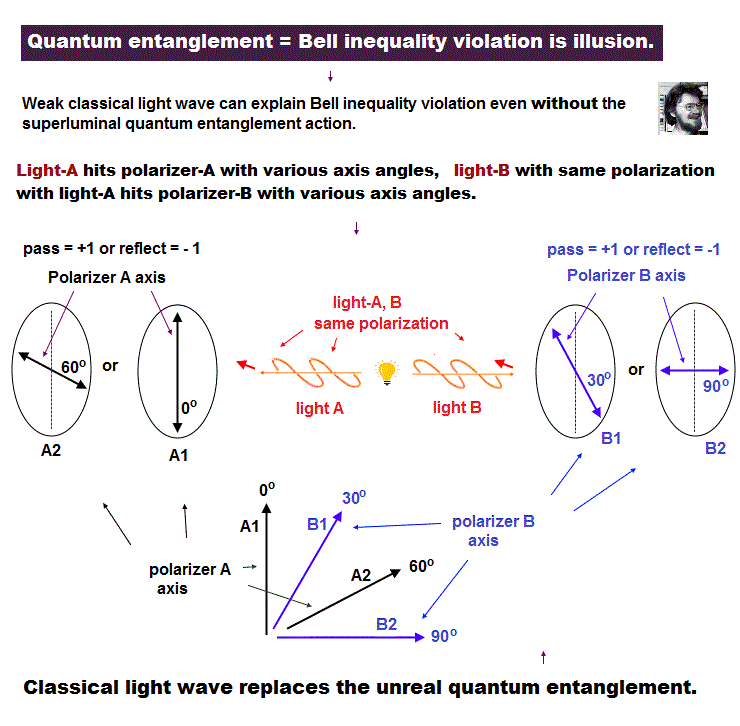Suppose, photon A bumps into two kinds of polarizing filters A1 whose axis points to 0 degree and A2 whose axis points to 60 degrees.

And photon B bumps into two filters B1 whose axis points to 30 degrees and B2 whose axis points to 90 degrees.

Fig.28 shows the axis directions in these four polarizing filters.
( Caution: The polarizing filters A1 and A2 are at the side of photon A, and the filters B1 and B2 are at photon B side.)

## Bell inequality ( CHSH type ) for proving fictitious superluminal entanglement action.

In this case, there are 16 patterns (= 24) in which photons (= light waves ) A and B pass (+) or are reflected (-) by the polarizing filters ( A1,A2,B1,B2 ), as follows,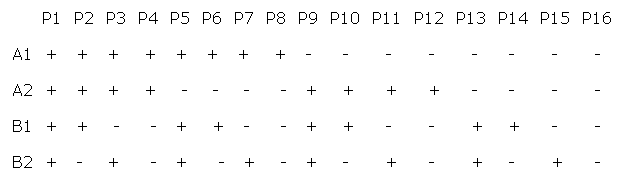where the sum of all these probabilities is 1 ( P1 + P2 + P3 + .....+ P15 + P16 = 1 ).
Here we define as follows,

---------------------------------------------------------------------
< A1, B1 > = ( the probability that the signs of A1 and B1 are the same, + + and - - ) - (the probability that the signs of A1 and B1 are different, + - and - + ) = (P1+P2+P5+P6+P11+P12+P15+P16) - (P3+P4+P7+P8+P9+P10+P13+P14)

In the same way,
< A2, B1 > = ( the probability that the signs of A2 and B1 are the same, + + and - - ) - (the probability that the signs of A2 and B1 are different, + - and - +) = (P1+P2+P7+P8+P9+P10+P15+P16) - (P3+P4+P5+P6+P11+P12+P13+P14)

< A2, B2 > = ( the probability that the signs of A2 and B2 are the same, + + and - - ) - (the probability that the signs of A2 and B2 are different, + - and - +) = (P1+P3+P6+P8+P9+P11+P14+P16) - (P2+P4+P5+P7+P10+P12+P13+P15)

< A1, B2 > = ( the probability that the signs of A1 and B2 are the same, + + and - - ) - (the probability that the signs of A1 and B2 are different, + - and - +) = (P1+P3+P5+P7+P10+P12+P14+P16) - (P2+P4+P6+P8+P9+P11+P13+P15)
---------------------------------------------------------------------

So we arrive at the following equation,

< A1, B1 > + < A2, B1 > + < A2, B2 > - < A1, B2 > = 2 ( K - L )
K = P1+P2+P6+P8+P9+P11+P15+P16
L = P3+P4+P5+P7+P10+P12+P13+P14

Using the relation, (K + L) = Σ Pi = 1,
-1 = - (K + L) ≦ (K - L) ≦ (K + L) = 1

So the following inequality (Bell inequality, CHSH type) must be satisfied in the fictitious indivisible photon particles with idealistically No photon loss (= which is impossible ),

| < A1, B1 > + < A2, B1 > + < A2, B2 > - < A1, B2 > | = 2 | K - L | ≦ 2

this p.2-3, this p.32-35,  this p.20-21,  this p.33-35,  this p.12

## Bell inequality violation = beyond classical limit "2" ?

For example, the probability that the results of the photons A (→ A1) and B (→ B1) are the same summing the cases of ( ++ = both photons A and B pass each polarizing filter ) and ( -- = both photons A and B are reflected from each polarizing filter ) is cos2 (A1-B1),
where (A1-B1) is the axis angle difference between polarizing filters A1 and B1 (= θ of Fig.20 ).

And the probability that the results are different summing ( + - = photon A passes and photon B reflects from each polarizing filter ) and ( - + = photon A reflects and photon B pass each filter ) is sin2 (A1-B1).

So, < A1, B1 > of Bell inequality is ( this p.4-10,  this p.3,  this p.2-right )
(Eq.1)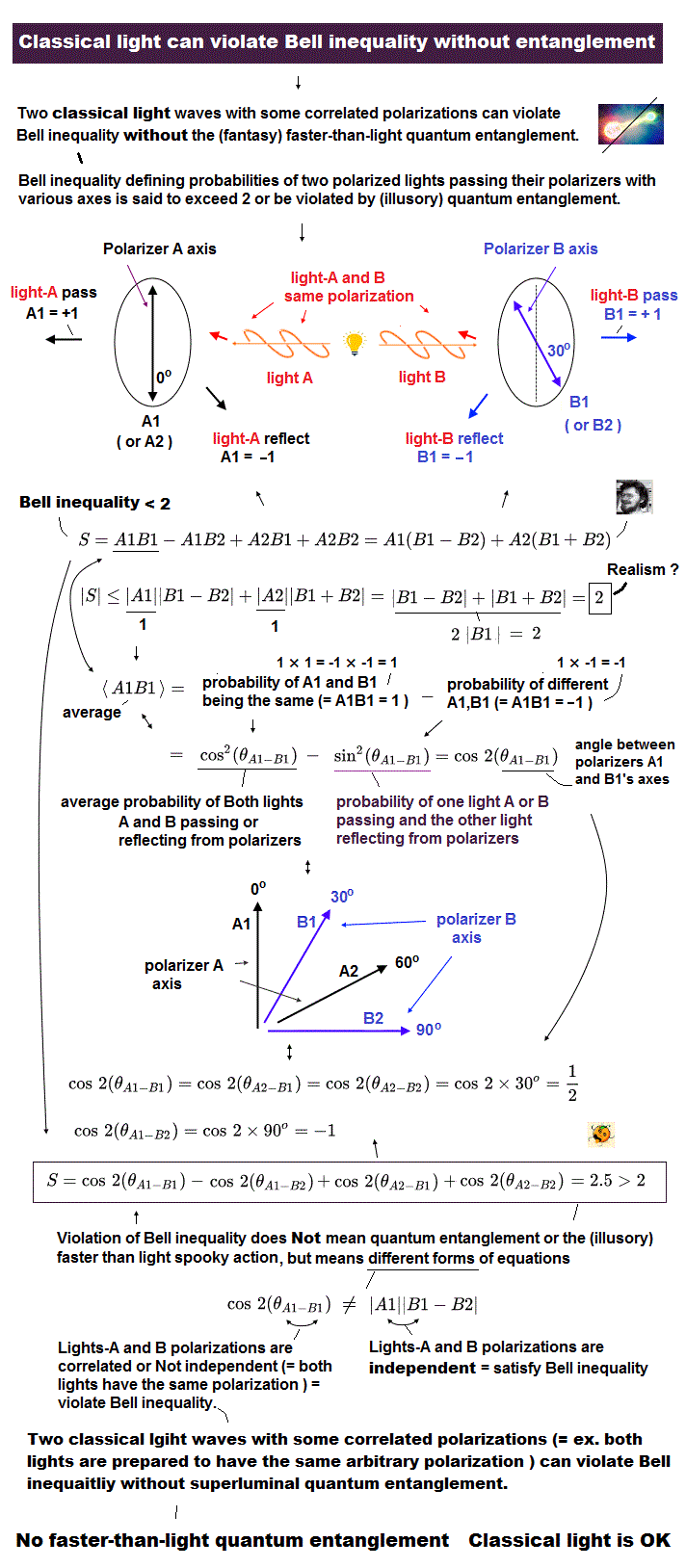where θA1 - B1 means the angle of (A1 -B1) between two polarizing filters's axes A1 and B1.

In the case of Fig.28, each cosine value becomes
(Eq.2)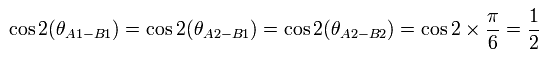and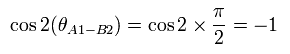From Eq.2, the above Bell inequality (CHSH type) is,
(Eq.3)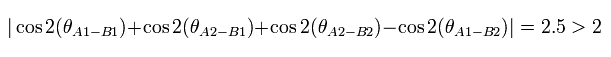This violates Bell inequality !

So physicists claim these two polarized photons A and B showed the faster-than-light quantum entanglement action, and we must give up any realities in quantum mechanics.  ← ridiculous.

But as I said, this illusory superluminal quantum entanglement is caused by falsely treating the realistic divisible classical light wave (+ detection threshold trick at the single photon detector ) as a fictitious indivisible photon particle.

## Detection "threshold" at photodetector (= Not fantasy superluminal entanglement link ) causes Bell inequatlity violation.

In conclusion, if the photon is a (fictitious) indivisible particle, the strange nonlocal phenomena such as the entanglement are actually occurring, and we must believe this fantasy faster-than-light transmission.

In other words, if the photon is a dividable electromagnetic wave, we need not consider this fantasy superluminal entanglement action.
You can easily judge which case (photon is a (fictitious) particle or realistic light wave ) is more natural.

## Detection loophole is Not closed, so quantum entanglement is illusion caused by the low coincidence detection efficiency of a pair of polarized photons (= or classical light wave )

In order to claim this unrealistic superluminal quantum entanglement link is true, physicists must detect two polarized photons simultaneouly with almost 100% coincidence detection rate, which is impossible ( this p.4-left-lower,  this p.22,  this p.3-3, Fig.2-3,  this p.3-Table.I,  this Fig.2, Table I ).

Physicists gave up using the fictitious photons and tried to conduct the illusory quantum entanglement experiments of high detection efficiency using two Beryllium ions (= Be+ ), arranging that when one Be+ ion's nuclear spin is up (or down ), the other Be+ ion spin is always up (or down,  = so up-up or down-down = entanglement !? No! ),  this p.2-3,  this p.1-left ).

↑ This Be+ ion case also has nothing to do with (illusory) superluminal quantum entanglement link, physicsts just artificially prepared two Be ions with the same-directional nuclear spins using the classical electromagnetic field and laser light so that both Be+ ions have the nuclear spins point in the same direction (= both Be+ ions are prepared to be in the same hyperfine energy levels ), which nuclear spin directions are estimated by illuminating Be+ ions with another lights ( this p.2 ) without using polarizing filters.

In the low detection efficiency photon case, physicists tried to change the original Bell inequality or intentionally avoid measureing the photons reflected from the polarizers to avoid the unsolvable issue of the intrinsically-low photon coincidence detection efficiency loophole ( this p.4,  this p.2-2nd-last paragraph, p.3-Table.I ).

↑ So the low detection efficiency problem (= low coincidence rate of counting both pass and reflect photons at each polarizing filter ) is Not solved, and they cannot exclude the classical light wave case.

As a result, the superluminal quantum entanglement is just illusion which can be naturally replaced by the realistic divisible classical light wave without the fantasy superluminal spooky action.

## Local realistic loophole ( using CHSH ) is NOT closed.

### [ It's about time that some top journal should change basic idea into "reality". ]

Because, they are obstructing development of "real" science under their present stance .First, all researchers and news writers should make efforts to explain to ordinary people to make them understand easily, and should NOT confuse them ONLY by fascinating words "entanglement".
It cannot be helped that some top journal had spreaded illusory fields such as entanglement and quantum computer due to the time gap related to three-body helium. ( I understand their feelings well. )
But aiming at illusory ideas such as closing loopholes forever, means we admit only unreality and "Shut up and calculate !" forever, which obstructs the development of science.

[ Bell inequality violation is "still" an illusion. ]In some website, we see the news about closing all local realistic ( fair sampling ) loophole.
But unfortunately, they did NOT close all loopholes by usual CHSH-type bell inequality.
As far as I see, different-type inequality allows lower-efficiency and some photon's loss.
And I cannot find all single (= not coincident ) events. ( They seem to use some limited single events, in inequality calculation. Is this really allowed ? )

Different from usual CHSH-type, this inequality relies ONLY on incomplete and Not enough informations = about a half of usual CHSH-type.
Anyway, this result cannot elimilate electromagnetic wave's local realistic model, as I explain above.
This different-type inequality experiment uses some light rotator, and NOT detect reflection from splitter.
In this method, our realistic electromagnetic wave model can give much more than 100 % efficiency (= their definition, coincident / single events ).

So this result cannot eliminate electromagnetic wave model.
( "Light rotator" uses phase change of a part of a photon, which means photon particle is just classical waves. )

[ All three loopholes have NOT been closed "simultaneously". ]You may read some articles such as all loopholes have been closed, so Einstein is wrong.
But this experiment closed only one loophole of "fair-sampling".
( The fair-sampling loophole is the only one left that hasn't been closed until now. )

So all three important loopholes of "detection", "locality" and "fair-sampling" loopholes have NOT been closed simultaneously.
This means spooky link has NOT been proved to be correct in any experiment.
I confirmed this fact in some site, but all webnews should explain this fact more clearly to ordinary people, I think.

"Freedom-of-choice" is important, because we can "artificially" choose the special situation which can violate Bell inequality. At least, they should violate usual CHSH inequality, not only in very special inequality.

[ Time gap causes various serious illusions and paradoxes. ]As I say, very complicated three-body helium couldn't be solved without computers.
Due to this time gap ( from 1920s to 1990s ), various illusory fields such as entanglement and quantum computers need to be created to fill this gap in physical world.
And serious paradoxes of special relativity have been hidden and eliminated from usual textbooks.

### [ Entangled "photons" at different times ? ]

(Fig.T-1) "1" and "4" photons can be really entangled at different times ?They claimed that recent experiment showed entangled "photons" that never coexisted in time.
But as I said many times, the trick of these kinds of experiments is they always use the word of "photon", NOT electromagnetic wave.

In Fig,T-1, entangled photon pair (= 1, 2 ) is generated at first BBO.
( One of them is horizontally polarized (= H ), and another is vertically polarized (= V). )
After detecting photon 1 state, another entangled pair of (= 3, 4 ) photons are generated.
Then, mixing photons 2 and 3, and they detect 2 and 3 photon states (= H or V polarizations. )
Of course, we cannot know which photon enters upper or lower detector, because they are mixed.

(Fig.T-2) Entangled photon pair is generated at BBO ?Entangled photon pair is often created by "parametric down-conversion" at BBO.
In this process, a photon of wavelength λ enters some crystal, and is split into a pair of photons of wavelength 2λ.
So the total energy is conserved. (= their frequency becomes half ).
But as I said many times, if photon is a particle, it cannot be split so easily.

And "horizontally" or "vertically" polarized states mean they are just classical electromagnetic waves ( NOT a particle ).
How can a point particle of photon be polarized ?? It's impossible.

(Fig.T-3) Pattern I   photons ( 2, 3 ) = ( V, V ) → ( 1, 4 ) = ( H, H ).When we detect both photons "2" and "3" as vertically polarized (= V ) photons, both photons 1 and 4 are automatically horizontally polarized photons (= H ).
It is quite natural, because they generated photon pair, one of which is H, and another is V at BBO.

So they insist photons 1 and 4 are in the mysterious "entangled" states.
But as you feel, these results are natural things from the usual classical light's viewpoint.
Then why they insist these states are faster-than-lihgt entanglement ?

(Fig.T-4) They suppose "virtual" classical "photon" pair,I don't think ordinary people understand why they consider these results as mysterious entanglement.
As I said in above section, these kinds of experiments assume, what we call, "classical" photons.
If classical photons really exists, they cannot be devided at beam spilitter, so they cannot always show the same polarization results at detector, even if their polarizations are in the same direction.

For example, in Fig,T-4, both filters A and B point in the same directions.
( And both photons A and B have the same polarization. )
In the entangled photons, when one of them passes the filter, another photon always passes the filter by spooky link.
But in classical photons, even when photon A passes the filter A, photon B does NOT always pass filter B, as shown in Fig,T-4 (= it reflects at the probability of sin2 α ).

(Fig.T-5) Both waves pass their filters ( ++ ) enough to be detected.If we think classical "photon" as illusion, and use the concept of classical "electromagnetic waves", we can easily explain these kinds of experiments from the local realistic viewpoint.

First, when we can detect both "2" and "3" photons at detectors, it means both "2" and "3" photons, which have passed the beam splitter, have strong light intensities enough to be detected as a "photon".
( If their intensities are weak, we cannot detect them as a single photon below threshold, and they are neglected. )

So in this case, both "1" and "4" also have strong light intensities, which are polarized in the specific directions, and we can detect them as "entangled" states, at the single photon detector (= H and H in this case ), because they are more than detection threshold.

For example, if you can detect photon "2" as "V", it means photon 2 almost points towards "V" before it enters beam splitter.
Because if photon 2 points between "V" and "H" (= 45 degree ) in advance, it is divided equally into pass and reflect directions ( cos2 45 = sin2 45 = 1/2 ), and cannot reach detection threshold.

(Fig.T-6) Pattern II.When we detect photons 2 and 3, as one is "V" and another is "H", it means one is H, another is V in 1 and 4 photons.
Again it is quite natural from the usual classical viewpoint.

"Bell state measurement" needs to detect both photons.
So if we can detect only one of 2 and 3 photons, their results are NOT used as experimental results. (= trick ! )
I'm sorry for experimentalists of entanglement, but if you spread "supernatural" phenomena such as faster-than-light entanglement, you should explain to ordinary people in more detail for them to understand your procedure well, I think.

## Entanglement of all particles (= atoms, spin1/2, hole ) are illusions.

### [ Why various top journals continue "Shut up and calculate !" about spin "forever" ? ]

Even in 2013 of 21th century, various top journals continue "Shut up and calculate !" about "spin".
( For example, the role of spin in organic photovoltaics, or quantum many-body spin system in an optical lattice clock even in 2013. )
As shown on this page, it is impossible that very weak spin magnetic moment can cause the energy difference between singlet and triplet states.

And S states (= angular momentum zero ) in each energy levels are impossible, too.
Because outer electron cannot penetrate many inner electrons and nucleus.

[ Doublet = spin or circular motion ? ]If the two electrons are entangled to the spin 0 state (spin up + spin down = spin 0), it is said that the magnetic moments are canceled out and become zero.
But this explanation is wrong. Because the two electrons are apart from each other by the repulsive Coulomb force. (If the two electrons having the opposite magnetic moments are completely sticking to each other, the sum of their magnetic moments becomes zero. But it is impossible.)

[ Electron spin is real ? ]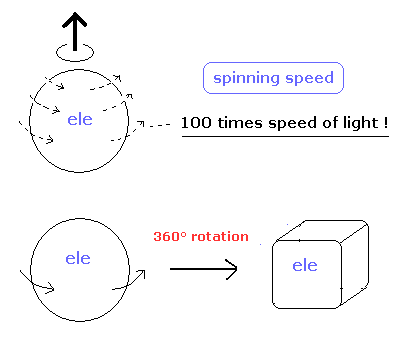And the spinning fermions don't go back to their original configurations by the 2π rotation. (By the 4π rotation, they return.)
And if the electron spin is caused by the real spinning of the electron, the electron rotation speed becomes more than 100 times faster than the speed of light.

So it is unnatural that we use this "mathematical" spinor for the "real" entanglement experiment (for example, like cosθ/2).
The original spin state may be changed by each experimental environment having some external magnetic fields. It is "artificial" manipulation

Bell inequality of spin 1/2 particles often appears in usual textbooks, though actual experiments treat other things such as photons.
Because this experiment with spin 1/2 particles is very difficult.
The important point is that the Bell inequality itself of spin 1/2 particle is "unreal" thing, which is a little different from that of photons.
We explain this reason later.

### [ Two neutral atoms really entangle each other ? ]

[ Rydberg blockage. ]It is said that they succeeded in entanglement of neutral atom-atom.
But as far as I see, these kinds of entanglement use imaginary (two) photons to detect them.
So we can explain this phenomenon by usual local classical mechanics ( NOT fantasied nonlocal ), as I said in above section.

When two Rb atoms are trapped and very close to each other (= several micrometers ), the energy to excite both two atoms is a little bigger due to dipole-dipole interactions.

[ Faster-than-light entanglement ? Really ?? ]So, they can excite only one of two atoms, and only this excited atom goes down to the lower hyperfine state (= red arrow ) by additional irradiation.
As a result, one of the two atoms is in the original upper hyperfine state, and another is the lower hyperfine state. (= 5s, upper and lower arrows. )
This state is an entanglement, they insist. But is it really so ?

To confirm these two hyperfine states, they use, what we call, (two) photons.
So at this moment, atom-atom entanglement is equal to photon- photon entanglement.
Furthermore, they just artificially make two atoms upper and lower hyperfine states, which is NOT related to entanglement.

### [ Electron hole entanglement is an illusion. ]

[ Faster-than-light entanglement ? Really ?? - part II. ]Basically, electron and hole, which are generated from each other, are correlated.
They often use very vague concept of "spin", this spin state can be measured by light polarization.
At this moment, spin-hole entanglement can be explained by classical lights, as I said above.
And spin has the same magnetic moment (= Bohr magneton ) as Bohr's orbit.

Or they insist the entanglement can be confirmed by measuring vague noise of thin insulator between them.
But as you see, the distance between electron and hole is so short, that we cannot confirm they are really faster-than-light.

Actually, these entanglement things are NEVER useful for our daily lives, though these kinds of experiments have been performed extremely many times.
These facts clearly proved the entanglement itself is just an illusion.

### [ Spin 1/2 entanglement is only a mathematical and imaginary thing. ]

First we define the next unit vector using the polar coordinates as follows,
(Eq.4)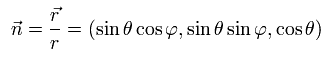When the spin points to the direction of this unit vector, the spin operator can be expressed as
(Eq.5)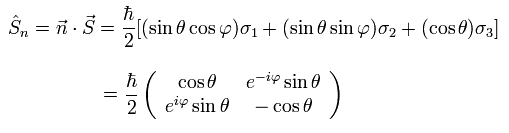This is an inner product of vectors n and S.

So the spinor of the eigenfunction, which points to the direction of n is
(Eq.6)And the spinor of the eigenfunction, which points in the opposite direction from n is
(Eq.7)Suppose two spin 1/2 particles of the opposite spins are flying in the opposite directions.
The spin of the particle A is measured by A, while the spin of particle B is measured by B.

First, we suppose the direction of measurement A points to the unit vector n of Eq.4.
If the spin of the particle A is +1/2 in the n direction at the measurement A, the particle B instantly becomes - 1/2 in the n direction. (= entanglement.)
This means that the instatnt the particle A becomes α of Eq.6, the particle B becomes β of Eq.7.

Next we suppose the direction of measurement B is z direction.
(So the angle betweem measurements A and B is θ.)
According to Eq.7, the probability that the spin of the particle B becomes -1/2 in the z direction is
(Eq.8)When the particle A is +1/2 at the measurement A and the particle B is -1/2 at the measurement B, this case is named "( + + )".
Because they are usually opposite from each other.

And the probability that the spin of the particle B becomes +1/2 in the z direction is
(Eq.9)When the particle A is +1/2 at the measurement A and the particle B is +1/2 at the measurement B, this case is named "( + - )".

In the same way, when the particle A is -1/2 at the measurement A, the particle A means β of Eq.7, and the particle B instantly becomes α of Eq.6.
So the probability that the spin of the particle B becomes +1/2 in the z direction is
(Eq.10)When the particle A is -1/2 at the measurement A and the particle B is +1/2 at the measurement B, this case is named "( - - )".

And the probability that the spin of the particle B becomes -1/2 in the z direction is
(Eq.11)When the particle A is -1/2 at the measurement A and the particle B is -1/2 at the measurement B, this case is named "( - + )".

So like the above photon case, the probability of ( + + ) and ( - - ) minus the probability of ( + - ) and ( - + ) is
(Eq.12)where θA1-B1 means the angle between the measurements A1 and B1.

Here we do the following four measurement (each particle has two). (Fig.29).

(Fig.29) Particle A → measurement A1 or A2. Particle B → B1 or B2.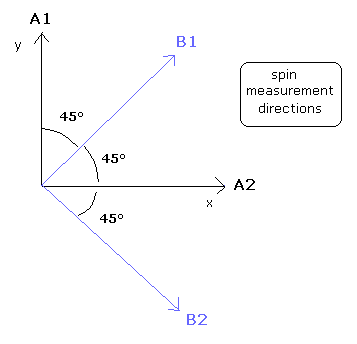So each cosine value can be calculated as (like Eq.12),
(Eq.13)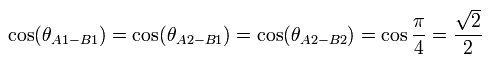and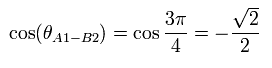So the Bell inequality of the spin 1/2 particle is
(Eq.14)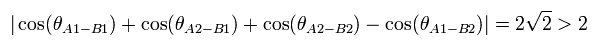This violates Bell inequality !

But we go back to Eq.6 and Eq.7 again.
According to Eq.6 and Eq.7, the spinor can't return by one rotation !
(It needs two rotations (= 720 degrees) for returning to its original form.)
So this spinor is NOT a real thing, either.
This means that Bell inequality itself of Eq.14 is wrong in the case of spin 1/2 particles.

As a result, it is very difficult to say that the "real" entanglement of spin 1/2 "real" particles is occurring.
( The illusions of Entanglements are caused by "unreal" particles such as photons and spin 1/2 particles.)

## All interpretations of QM such as MWI, Copenhagen and BM are meaningless ?

The many worlds interpretation(MWI) was proposed first by Hugh Everett .
And now the MWI is considered as the best interpretation of QM.
But many people including I think it is only fantasy.

In many-worlds, the collapse of the wavefunctions in superposition states is explained by the mechanism of quantum decoherence.
By decoherence, many-worlds claims to resolve all of the correlation paradoxes of quantum theory, such as the EPR paradox and the Schrödinger's cat.
(But I think "decoherence" is very intentional and a very convenient way to interpret.)
In MWI, there is no strange observation-triggered wavefunction collapse which the Copenhagen interpretation proposes.
(Surely, it is unnatural that we treat only "observer" as a "special" thing.)

(Fig.30) All interpretations are unnatural ? → "Shut up and Calculate !"The most "real" interpretation of the quantum mechanics is the Bohmian mechanics (BM) ( De Broglie - Bohm theory or pilot wave ).
But strange to say, the BM electron doesn't have the charge and spin 1/2.
Because if the BM electron has the charge, it radiate energy as it is moving.

On the other hand, in the Bohr model, when the orbital length is a integer times the de Broglie's wavelength, the electron's motion becomes stable not radiating the energy.
The BM obeys the probability density of the Schroedinger equation, so the electron's movement is much more complex than the Bohr's model.
And strange to say, the probability density near the point at infinity of the ground state hydrogen electron is not zero.

More important problem is that all these interpretations are inconsistent with the relativistic quantum field theory(QFT).
All interpretations of QM are based on the Schroedinger equation(S.E.) which is acausal and "probabilistic".
But the nonrelativistic S.E. can't explain the relativistic effects including the fine structure (spin-orbital interactions) .

As the atoms become heavier (which means the atomic nucleus charge becomes larger), the experimental results become more different from that of S.E., because the electon's speed becomes faster. In S.E., irrespective of the nucleus charge, the electrons of any atoms are all static as electron clouds obeying the probability density.
So the Schroedinger equation is incomplete in explaining the experimental results..

On the other hand, the Dirac equation (D.E.) which satisfies the (relativistic) causality can explain this relativistic effects.
Of course, D.E. is not "probabilistic".(= D.E. doesn't show the probability density.) So the causal Dirac equation can be said to be only a "mathematical" model.
So the relativistic QFT is inconsistent with all interpretations and the nonlocal phenomena such as the entanglement.
This means the QM contains self-inconsistency.

And according to "superposition" of the QM, the Benzene's electrons are in the many-world like superposition state !
From the classical viewpoint, the Benzene's electrons are only oscillating and moving.
So the strange "superposition" state can be replaced by the classical movement "naturally".

How about the Bohr-Sommerfeld model ?
Sommerfeld developed the original Bohr model to the model which can explain the relativistic effect completely, (which result accidentally coincided with that of the Dirac hydrogen model).
Of course, the Bohr-Sommerfeld model dosn't contain self-inconsistency at all.

## References :

 A. Einstein, B. Podolsky, N. Rosen: "Can quantum-mechanical description of physical reality be considered complete?" Phys. Rev. 41, 777 (1935).

 J. Bell: "On the problem of hidden variables in quantum mechanics" Reviews of Modern Physics 38 #3, 447 (1966).

 A. Aspect, Dalibard, Roger: "Experimental test of Bell's inequalities using time-varying analyzers" Phys. Rev. Letters 49 #25, 1804 (1982).

 A. Aspect, P. Grangier, G. Roger: "Experimental realization of Einstein-Podolsky-Rosen-Bohm gedanken experiment; a new violation of Bell's inequalities" Phys. Rev. Letters 49 #2, 91 (1982).

 Hugh Everett: "Relative State Formulation of Quantum Mechanics" Reviews of Modern Physics 29 454 (1957).

Leggett Teleportation Cheshire cat (14/8/28) Delayed choice quantum eraser. Quantum computer (14/4/13).2022/10/6 updated. Feel free to link to this site.